### Home > CALC > Chapter 1 > Lesson 1.2.5 > Problem1-84

1-84.
1. Examine the graph of the function f(x) = 0.5x − 2 below. Homework Help ✎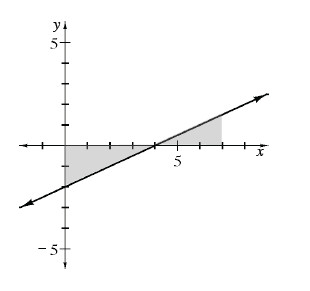1. Find the area of the shaded region using geometry. (Recall that the area below the x-axis is considered negative.)

2. Find k if A(f, 0 ≤ xk) = l0. How did you find your solution?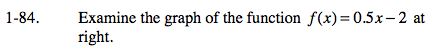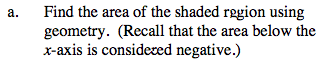Find the area of each triangle separately. Recall that the area belowthe x-axis is negative. Add the negative and the positive regions together.

[Area of triangle A] + [Area of triangle B]

$\frac{1}{2}(4)(f(0))+\frac{1}{2}(7-4)(f(7))$

$\frac{1}{2}(4)(-2)+\frac{1}{2}(3)(1.5)=$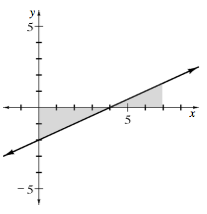$-1\frac{3}{4}$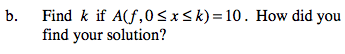[Area of triangle A] + [Area of triangle B] = 10

Simplify, and solve for k.